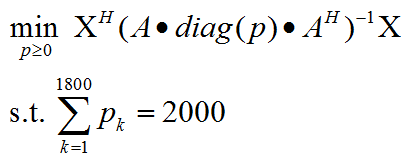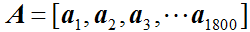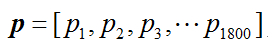# What is the problem with this?how to solve?

cvx_begin
variable p0(1801,1)
subject to
norm(p0,1)=norm(X,2)^2/10,p0>=0
cvx_end

Error using evalin
Undefined function ‘inv’ for input arguments of type ‘cvx’.

Error in minimize (line 14)
x = evalin( ‘caller’, sprintf( '%s ', varargin{:} ) );

Error in dan2019522 (line 45)

`inv` can not be applied to a CVX variable or expression. it is not listed in http://cvxr.com/cvx/doc/funcref.html#built-in-functions or http://cvxr.com/cvx/doc/funcref.html#new-functions

However, in the latter link you will see `matrix_frac` .

help matrix_frac

matrix_frac Matrix fractional function.
matrix_frac(x,Y), where Y is a square matrix and x is a vector of the
same size, computes x’*(inv(Y)*x) if Y is Hermitian positive definite, and
+Inf otherwise.

``````  An error results if Y is not a square matrix, or the size of
the vector x does not match the size of matrix Y.

Disciplined convex programming information:
matrix_frac is convex and nonmonotonic (at least with respect to
elementwise comparison), so its argument must be affine.
``````

Also, `norm(p0,1)=norm(X,2)^2/10,p0 >=0` doesn’t make any sense. I can;t tell you how to fix it because I don’t know what you’re trying to do.

I have tried matrix_frac，but it is useless.
inv is a function that comes with matlab and it means matrix inversion.I have used it in another cvx problem and it is no problem.
The original problem is:Everything in the objective function other than p is input data.
X and a is 10 * 1 vector.
A is 10 * 1800 array.`minimize(matrix_frac(inv(A)*X,diag(p)))`

`inv` is a “problem” if applied to a CVX variable or expression; it will produce an error message. `inv` can be applied to input data (i.e., constant from CVX’s perspective), as used in the code shown here.

I have used what you said.That line is not wrong.But A new problem has arisen.

cvx_begin
variable p(1801,1)
minimize(matrix_frac(Ani*X,diag§))
subject to
norm(p,1)==norm(X,2)^2/10,p>=0
cvx_end

``````Error using cvxprob/newcnstr (line 192)
Disciplined convex programming error:
Invalid constraint: {convex} == {real constant}

Error in cvx/eq (line 12)
b = newcnstr( evalin( 'caller', 'cvx_problem', '[]' ), x, y, '==' );

Error in dan2019522 (line 48)
norm(p,1)==norm(X,2)^2/10,p>=0
``````

The original problem is a SDP problem.Then I make a change to program:

cvx_begin
variable p(1801,1)
minimize(matrix_frac(Ani*X,diag§))
subject to
norm(p,1)<=norm(X,2)^2/10,p>=0
cvx_end

And SDPT3 solver is called.But the result is obviously wrong.The cvx_status is ‘failed’.I just want to make sure the sum of p is a constant that i define.
Thank you

I now see that you are trying to include two constraints on one line, separated by a comma.

Your first attempt, `norm(p0,1)=norm(X,2)^2/10`, is an illegal expression assignment.attempt.

Your second attempt, `norm(p0,1) == norm(X,2)^2/10` is a nonlinear equality constraint, which is non-convex and violates CVX;s DCP rules, hence the error message you received.

The last version you show, `norm(p,1) <= norm(X,2)^2/10` is convex and acceptable to CVX. it is different than the non-convex equality constraint.

The sum of p can be constrained to be a constant with the constraint `sum(p) == your_constant`. DO you also want a constraint on the *one) norm of p? If so `norm(p,1) <= some_constant` is convex and allowed; while norm(p,1) == some_constant is non-convex and is not allowed in CVX.

If you really want the non-convex norm equality constraint, either use a tool which allows non-convex constraints, or try the approach provided in @stephen_boyd’s answer at How to handle nonlinear equality constraints?